Integrable system

(diff) ← Older revision | Latest revision (diff) | Newer revision → (diff)
A differential system of dimension(cf. Involutive distribution) on an-dimensional differentiable manifoldthat has, in a neighbourhood of each point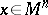, an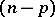-parameter family of-dimensional integral manifolds (cf. Integral manifold). One often speaks of a totally-integrable system in this case; more precisely it is defined as follows. Suppose that at each pointa subspace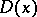of dimensionin the tangent spacehas been distinguished, such that a differential system, or distribution,of class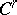,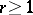, of dimensionis given on. The systemis called totally integrable if for each pointthere is a coordinate system,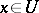,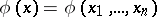, such that for any constants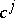,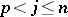, the manifold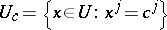is an integral submanifold, i.e. its tangent space at an arbitrary point coincides with. For analytic conditions that are necessary and sufficient for this, see Involutive distribution.
Cf. also Pfaffian equation. The phrase integrable system is also used to refer to a completely-integrable Hamiltonian system or equation, i.e. a Hamiltonian equation (system) on a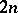-dimensional phase space which has(including the Hamiltonian itself) integrals in involution, cf. Hamiltonian system.